JavaScript和ABAP的尾递归

+关注继续查看

Before we start to research tail recursion, let’s first have a look at the normal recursion.

A simple factorial implementation by recursion: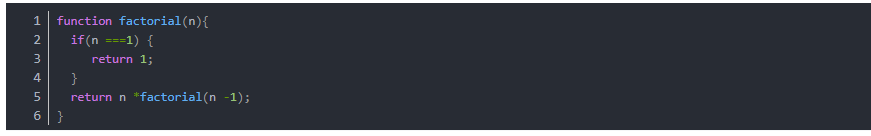Let N = 5, see how new stack frame is created for each time of recursive call: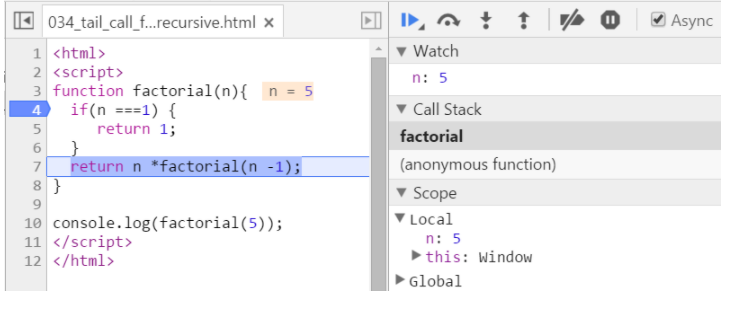We have two stack frames now, one stores the context when n = 5, and the topmost one for current calculation: n = 4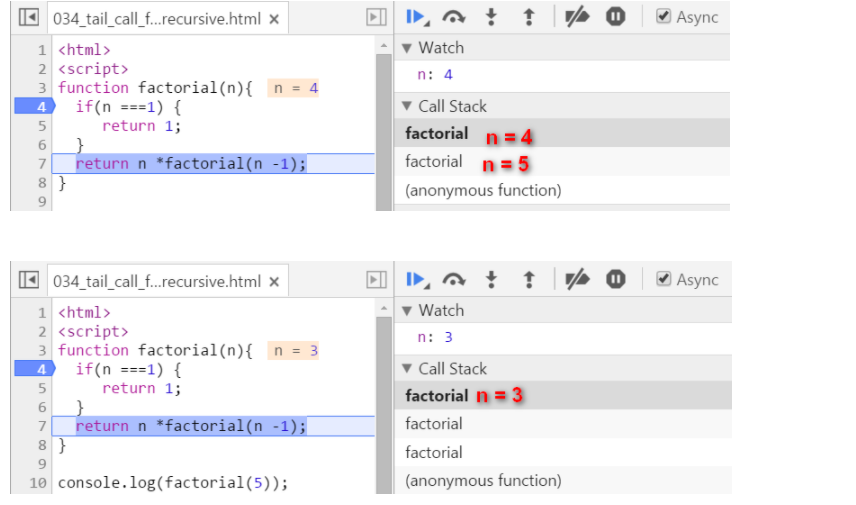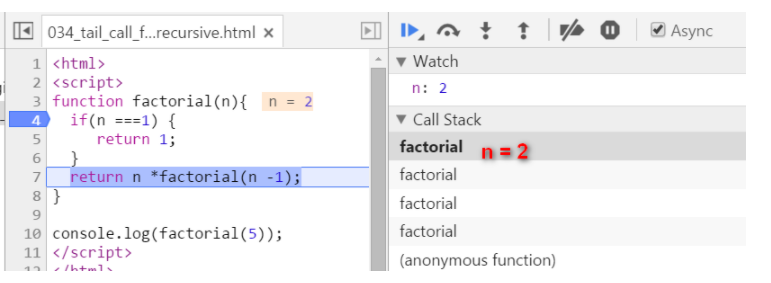Now since n equals to 1, we stop recursion. The current stack frame ( n = 1 ) will be poped up, the frame under it will be activated and become the topmost frame, with calculated result 1 passed into.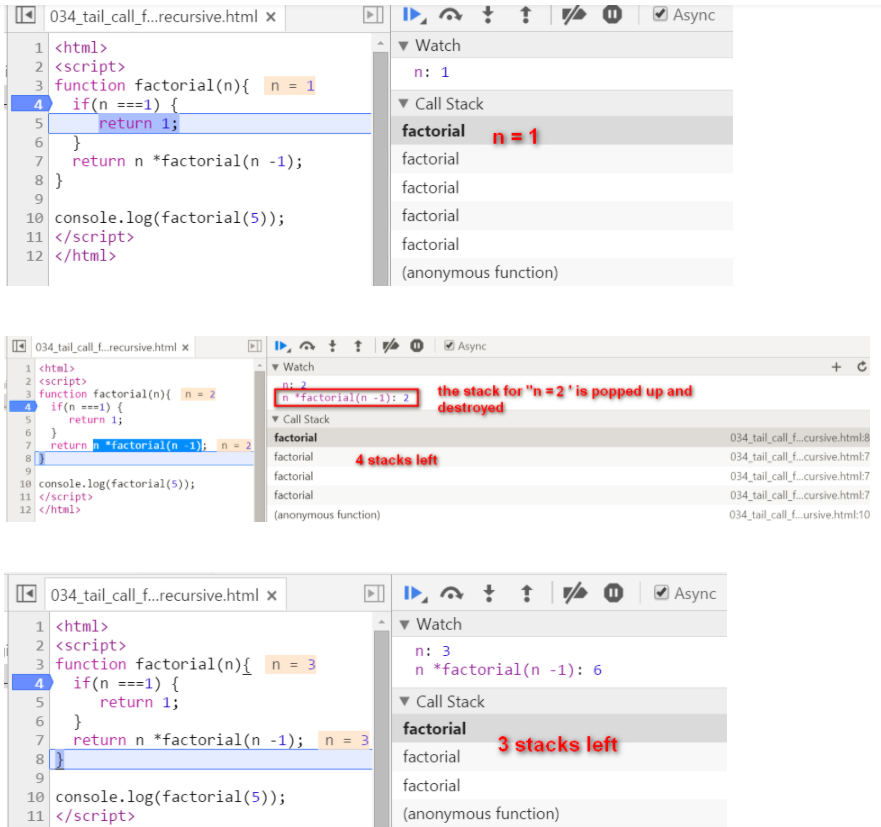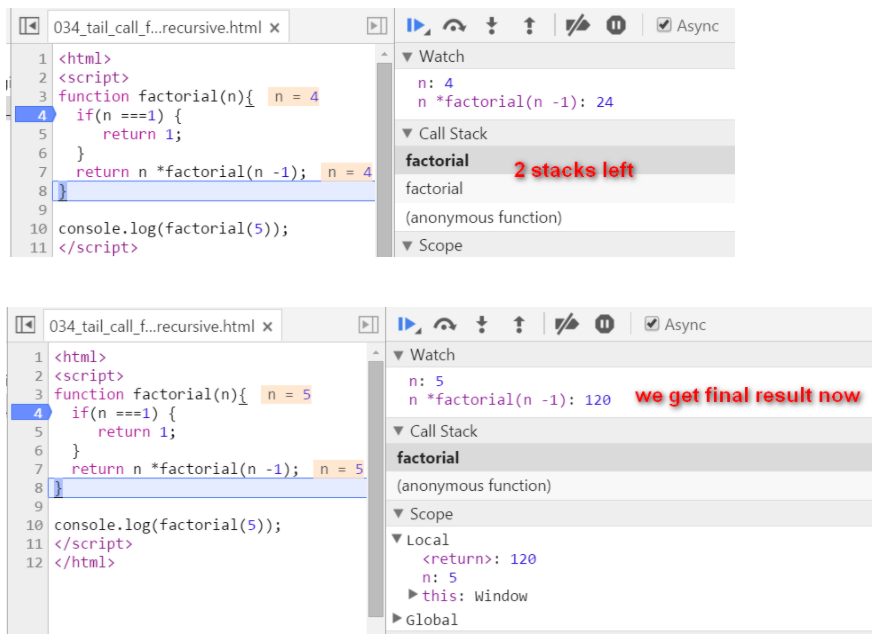key note for normal recursion: during recursion, every generated stack frame is needed and could not e destroyed until the final result is calculated. The calculation is actually not started until the recursion reaches the end ( the condition n === 1 fulfills ). If N is a big integer, it will lead to huge number of stack frames and finally the “stack overflow” or “out of memory” is inevitable.

tail recursion

Source code below: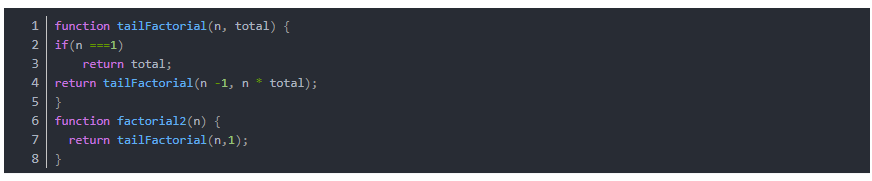There are two biggest differences compared with normal recursion:

(1) A new internal function tailFactorial is introduced here.

(2) The calculation is actually now spread within every recursive stack frame. Each frame finishes one part of calculation and pass the current result to the next frame. Once the current stack frame finishes its task, it is actually not needed any more. And thus for example the model browser can then do some optimization on those useless stack frames.

Observe the stack frame for tail recursion step by step: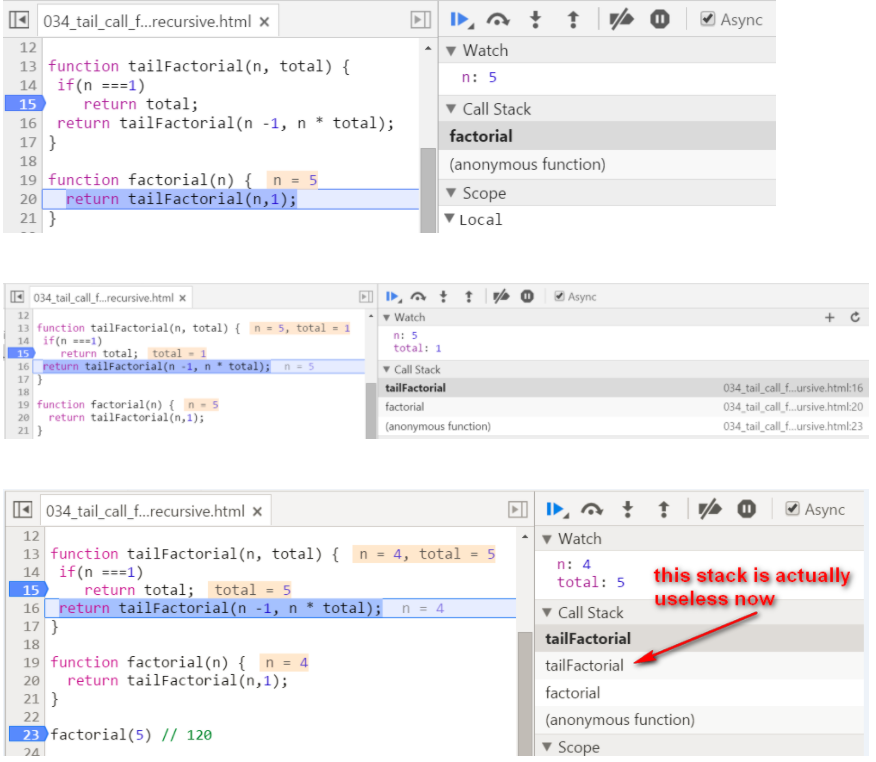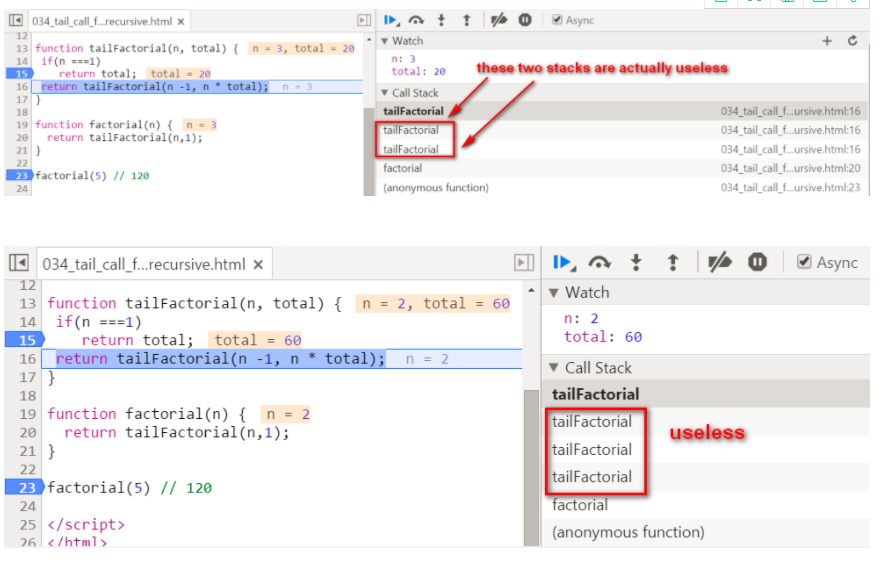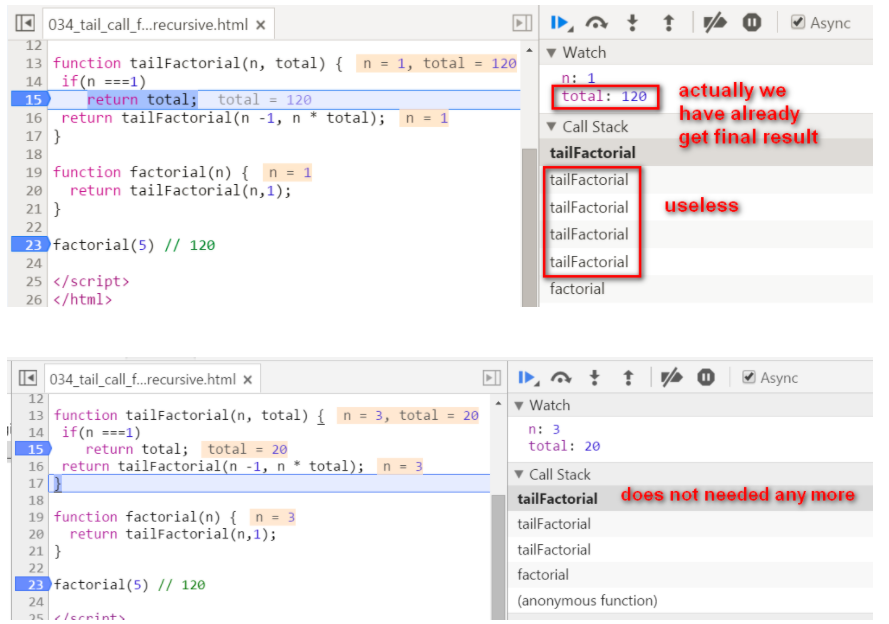stack popped up: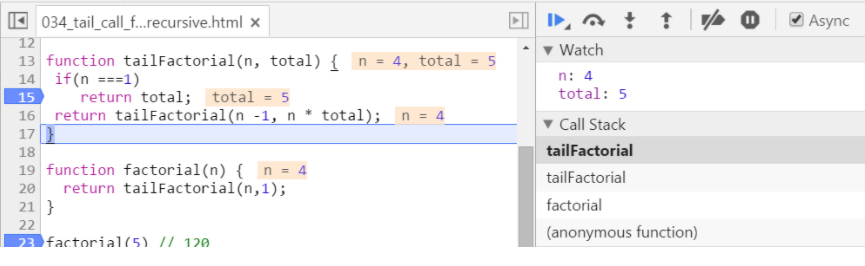When N = 20, the tail recursion has a far better performance than the normal recursion: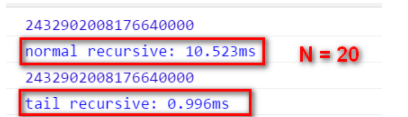Update 2016-01-11

Tail recursion implementation via Scala: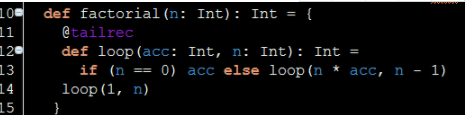The interesting thing is, after the Scala code is compiled into Java Byte code, compiler will eliminate the recursion automatically: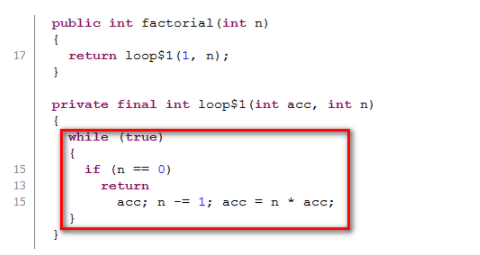Tail Recursion in ABAP

First this is the normal recursion: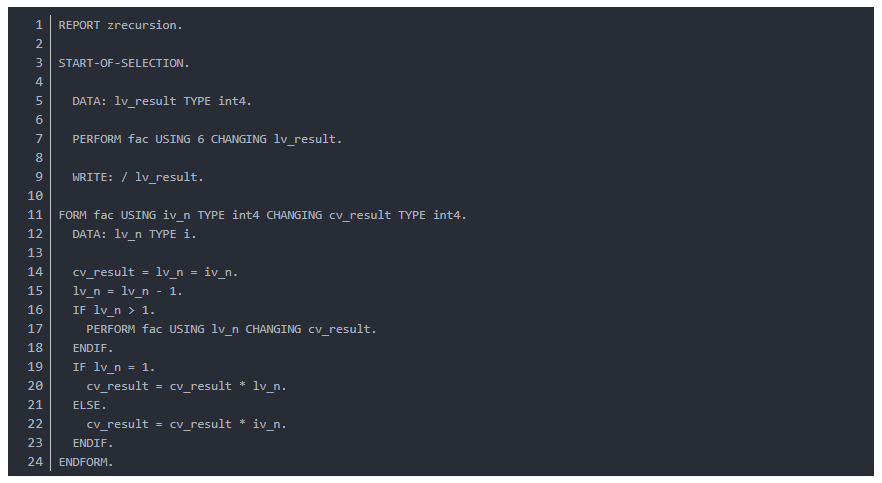And here comes tail recursion version: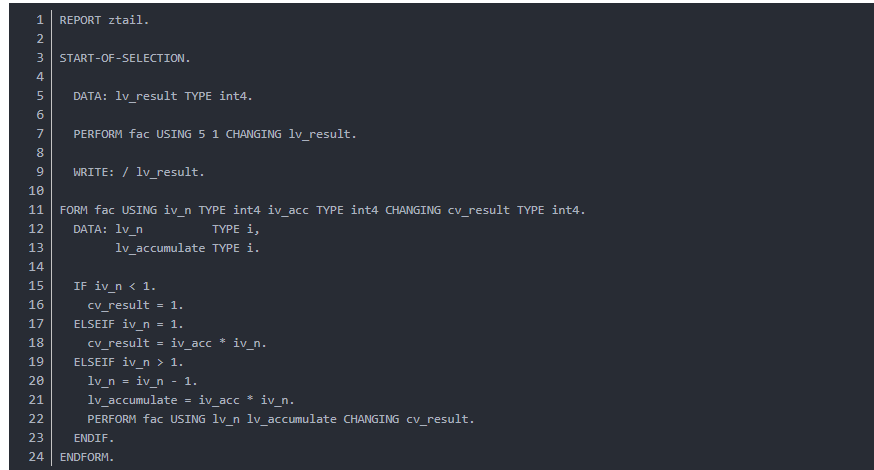10099 06767 014503 0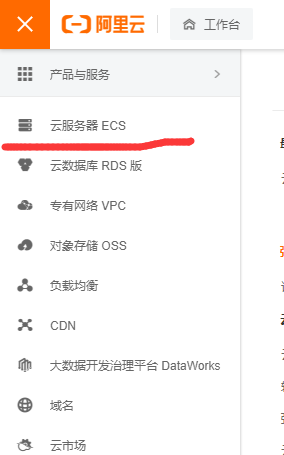2512 013897 0windows server 2008阿里云ECS服务器安全设置

9161 0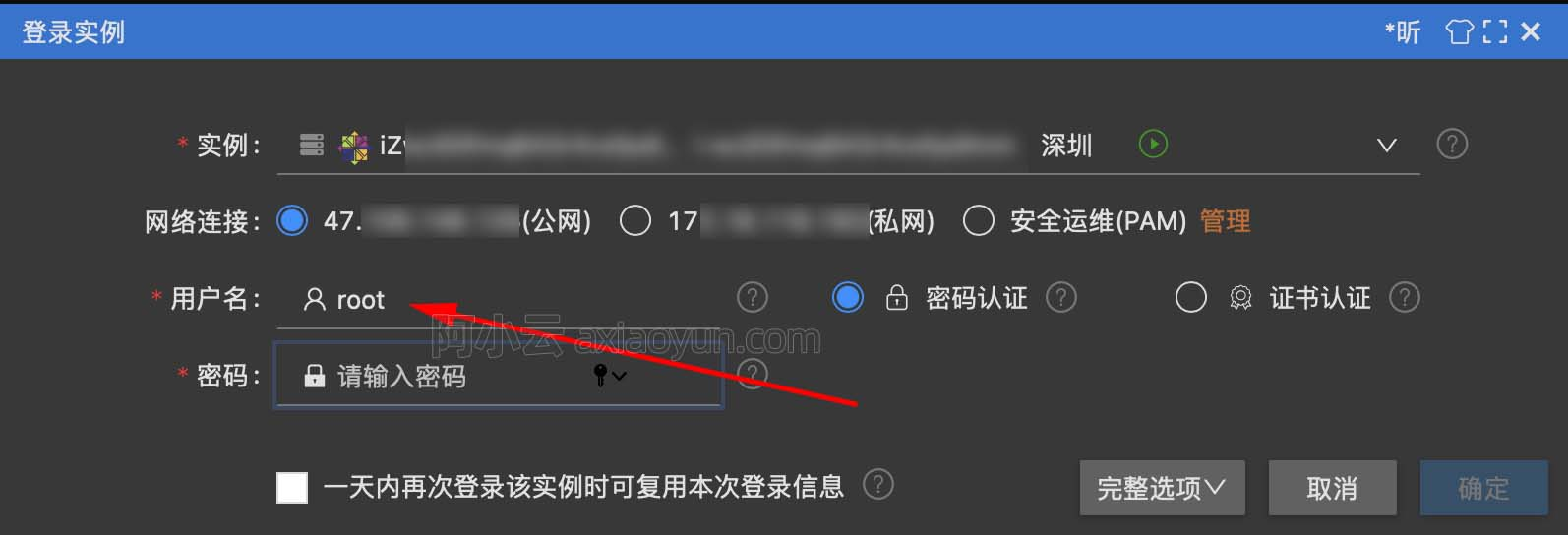4511 022415 054770 07366 0+关注
2628

0

+ 订阅

《2021云上架构与运维峰会演讲合集》

《零基础CSS入门教程》

《零基础HTML入门教程》